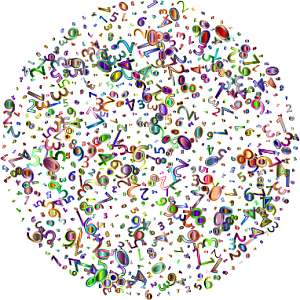# Prime Factorization

Instructions: Use this prime factorization to compute the factors and prime decomposition of a given number you provide in the box below.The number you want the factors of (Any positive integer number)

## How to use this prime factorization calculator

This calculator will provide you with the factors and the corresponding prime decomposition of a given number. So, you need to provide a valid integer, a positive integer.

Then, once that is provided, you need to click on "Calculate", in order to get all the steps of the calculation shown.## How do compute the prime decomposition

All you need to do is to find the factors of the corresponding number. These factors are then grouped there will be an exponent associated to each of them (reflecting the number of times the corresponding prime appears in the factorization).

## What are the steps for a prime factorization

• Step 1: Identify the number you want to factor. It must be a positive integer, otherwise you cannot proceed
• Step 2: Find ALL the factors of the number
• Step 3: Count the number of times each factor appears in the decomposition

## Why would need to deal with prime numbers?

Although they are not really covered in basic Algebra, prime numbers play a crucial role in Mathematics, not only Algebra. It seems that primes hold some sort of magic power and they have some incredible properties.

At the basic level, let's consider the fact that every single positive integer admits one and only one prime decomposition to be a sufficiently important property.### Example: Calculating a prime decomposition

Calculate prime factorization of 3468.

Solution:

First, we need to find all the possible prime divisors of $$n = 3468$$. In this case, it is found that

$3468 = 2\cdot2\cdot3\cdot17\cdot17$

Now, grouping the divisors found above, the following prime decomposition is obtained, in exponential form:

$3468 = 2^2\cdot3\cdot17^2$

This completes the process of the prime decomposition calculation, because no factors can be further decomposed.

### Example: Another prime number

Find the factors of 16.

Solution:< First, we need to find all the possible prime divisors of $$n = 16$$. In this case, it is found that

$16 = 2\cdot2\cdot2\cdot2$

Now, grouping the divisors found above, the following prime decomposition is obtained, in exponential form:

$16 = 2^4$

This completes the process of the prime decomposition calculation, because no factors can be further decomposed.

### Example: Another prime number

Find the factors of 137.

Solution: We need to find all the possible prime divisors of $$n = 137$$. In this case, it is found that the number $$n = 137$$ does not have any factors, and therefore, it is prime, hence, the prime decomposition of $$n = 137$$ is itself.

This completes the process of the prime decomposition calculation, because no factors can be further decomposed.

### Example: Prime decompositions and primes

Is 341 a prime number?

Solution: First, we need to find all the possible prime divisors of $$n = 341$$. In this case, it is found that

$341 = 11\cdot31$

Since there is a factor (11) that is neither 1 nor 341, we conclude that 341 is prime.

## More algebra calculators

Algebra is a very important branch of mathematics, perhaps the most important since it provides the basic grounds for most of the other math fields.

In terms of algebra calculators, you may be interested in computing general algebraic expressions, or also in terms of computing the least common multiple between two numbers, just to mention a few.